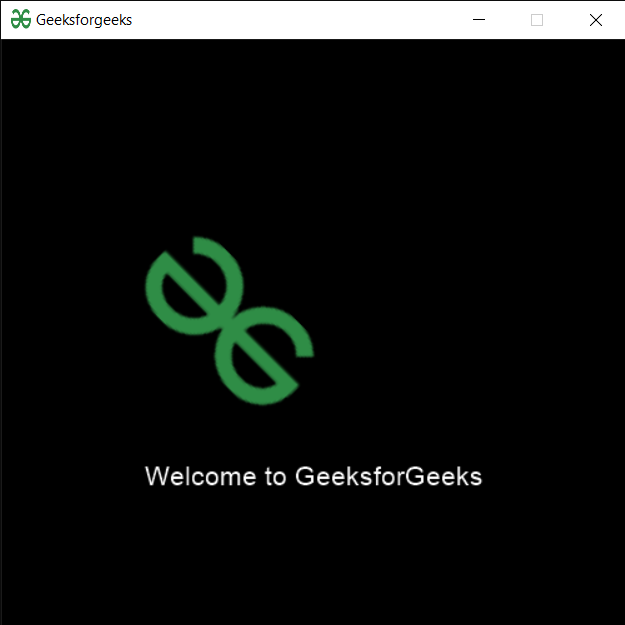# PYGLET – Updating Sprite

In this article we will see how we can update the sprite in PYGLET module in python. Pyglet is easy to use but powerful library for developing visually rich GUI applications like games, multimedia etc. A window is a “heavyweight” object occupying operating system resources. Windows may appear as floating regions or can be set to fill an entire screen (fullscreen). A sprite is an instance of an image displayed on-screen. Multiple sprites can display the same image at different positions on the screen. Sprites can also be scaled larger or smaller, rotated at any angle and drawn at a fractional opacity. Image is loaded with the help of image module of pyglet. Updating means to changing the properties of sprite, properties can be size, position etc.
We can create a window and sprite object with the help of commands given below

```# creating a window
window = pyglet.window.Window(width, height, title)

# creating a sprite object
sprite = pyglet.sprite.Sprite(img, x, y)```

In order to create window we use update method with sprite object
Syntax : sprite.update(x=None, y=None, rotation=None, scale=None, scale_x=None, scale_y=None)
Argument : It takes optional argument i.e x, y co-ordinates (int), rotation (float), scale (float), horizontal scale (float), vertical scale (float)
Return : It returns None

Below is the implementation

## Python3

 `# importing pyglet module ` `import` `pyglet ` `import` `pyglet.window.key as key` `  `  `# width of window ` `width ``=` `500` `  `  `# height of window ` `height ``=` `500` `  `  `# caption i.e title of the window ` `title ``=` `"Geeksforgeeks"` `  `  `# creating a window ` `window ``=` `pyglet.window.Window(width, height, title) ` `  `  `# text  ` `text ``=` `"Welcome to GeeksforGeeks"` ` `  `# creating label with following properties` `# font = cooper` `# position = 250, 150` `# anchor position = center` `label ``=` `pyglet.text.Label(text, ` `                          ``font_name ``=``'Cooper'``, ` `                          ``font_size ``=` `16``, ` `                          ``x ``=` `250``,  ` `                          ``y ``=` `150``, ` `                          ``anchor_x ``=``'center'``,  ` `                          ``anchor_y ``=``'center'``)`     `# creating a batch ` `batch ``=` `pyglet.graphics.Batch()`   `# loading geeksforgeeks image` `image ``=` `pyglet.image.load(``'gfg.png'``)`       `# creating sprite object` `# it is instance of an image displayed on-screen` `sprite ``=` `pyglet.sprite.Sprite(image, x ``=` `200``, y ``=` `230``)` `  `  `# on draw event ` `@window``.event ` `def` `on_draw(): ` `      `  `    ``# clear the window ` `    ``window.clear() ` `      `  `    ``# draw the label` `    ``label.draw() ` `    `  `    ``# draw the image on screen` `    ``sprite.draw()` `      `  `# key press event     ` `@window``.event ` `def` `on_key_press(symbol, modifier): ` `  `  `    ``# key "C" get press ` `    ``if` `symbol ``=``=` `key.C: ` `        `  `        ``# printing the message` `        ``print``(``"Key : C is pressed"``)` `        `  `# image for icon ` `img ``=` `image ``=` `pyglet.resource.image(``"gfg.png"``) `   `# setting image as icon ` `window.set_icon(img) `   `# updating the sprite` `# x, y = 10, 300` `# rotation = 45` `# scale = 2` `sprite.update(``100``, ``300``, ``45``, ``2``)`     `   `  `# start running the application ` `pyglet.app.run() `

Output :Whether you're preparing for your first job interview or aiming to upskill in this ever-evolving tech landscape, GeeksforGeeks Courses are your key to success. We provide top-quality content at affordable prices, all geared towards accelerating your growth in a time-bound manner. Join the millions we've already empowered, and we're here to do the same for you. Don't miss out - check it out now!